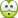# Term: Neutral Buoyancy

anorlunda
Staff Emeritus
Hi. I don't match your result of 9800 seconds. Can you please show how you got your result.

Chet
I'll post the calculation tomorrow.

Post #16.Pardon me. I missed #16. Your did indeed seem to hit the nail on the head.

Pardon me. I missed #1
No pardon necessary. I am happy someone else went down the same road and saw similar stuff.haruspex
Homework Helper
Gold Member
The stability of a submerged object is a separate issue. In order to remain stable while submerged, the center of buoyancy of the vessel must always be lower than the center
Off topic I know, but I think you have that backwards. The centre of buoyancy needs to be above the centre of gravity for stability.
(I've heard this also quoted in reference to buoyancy of surface vessels, but it is not correct there. The cross-sectional profile also matters in that case.)

anorlunda
Staff Emeritus
Sorry for the delay, this week was hectic. I promised to post how I calculated the 9800 second time constant in #21. This time around I used a different approach and came up with a different number. However, the conclusion stands that even a 0.01% change in water density is material.

• The starting point is Newton’s 2nd law ##f=ma##, plus Chet’s calculation that the water density changes 0.01% from ##y= 5\ meters## depth to ##y=20\ meters##depth. Viscosity and friction are not specifically addressed because the velocities are so slow.
• First I note that the ratio ##\frac{f}{m}## for buoyant forces is the same for small objects as large ones. So with scale independence, I simply choose m=1 kg.
• The problem is, given a 1kg submarine neutrally buoyant at 5 meters depth, push it down to 20 meters depth and release. What happens then? (An aside: we could also be analyzing debris which floats below the surface, but does not sink to the bottom, nor rise to the surface. I live on the water and see such objects from time to time and I know how they behave.)
• If we integrate acceleration twice to get position, then put a negative feedback loop around it, we have described a harmonic oscillator. Real life experience contradicts that, so I am arbitrarily asserting that the object will return to 5 m with a transfer function ##\frac{1}{1+\tau s}##. The step response is ##1-e^{-\frac{t}{\tau}}##. Given the specifics of this problem, depth y as a function of time becomes ##20-(1-e^{-\frac{t}{\tau}})(20-5)=y##.
• Assume that the initial acceleration is constant ##a=\frac{f}{m}=\frac{10^{-4}}{9.8}## where ##10^{-4}## is 0.01% and 9.8 is required to convert ##kg_f## to newtons. The time to rise the first meter (from 20 to 19) is ##t=\sqrt{2\cdot9.8\cdot10^4}=443\ seconds##.
• Here’s the approximation. Combine the y versus time behavior assumed with the calculated time to rise the first meter with constant acceleration. Thus we evaluate the depth using t=443 and y=19 which becomes ##\left[20-(1-e^{-\frac{443}{\tau}})(20-5)=19\right]## , and solve for ##\tau##. We get ##\tau=1985\ seconds##.
So 1985 seconds is my new estimate for tau.(Unless I made calculation errors which is something I do frequently. It has been 60 years since I was a student working problems.) It depends on three assumptions that are admittedly a bit of hand waving but good enough for rough estimates.

1. That the behavior of the object is to respond to step changes like a simple time constant, rather than like a harmonic oscillator.

2. That the time constant value can be approximated by the behavior for the first 1 meter rise out of 15, and that rise is characterized by constant acceleration.

3. That the submarine object is incompressible.

Of course, the numerical value of ##\tau## is not the point. As I said in #21, the only material point in this thread's question about neutral buoyancy is the differential compressiblity.

Chestermiller
Mentor
I don't know how you got an acceleration of ##10^{-4}/9.8##, but I get an initial acceleration of ##9.8\times 10^{-4}## from the equation:$$ma=0.0001mg$$. If x is the depth (in meters) below 5 meters, the acceleration at any time will be (0.0001)(9.8)x/15. So, we have:$$\frac{d^2x}{dt^2}=-(0.0001)(9.8)\frac{x}{15}$$. The solution to this equation under the initial condition that x = 15 at t = 0 and dx/dt = 0 at t =0 is: $$x = 15\cos(0.008t)$$According to this result, x will reach a value of 0 (sub will reach 5 meters) at 0.008t=π/2. This comes out to t =196 seconds. If we neglect the fact that the acceleration is decreasing as the sub rises, then we get ##t=\sqrt{\frac{(2)(15)}{(0.0001)(9.8)}}=175 ## seconds. My numbers for t are about 10x smaller than yours because of the location of the 9.8 in the numerator vs the denominator of the acceleration. This is why I was questioning your results in that earlier post.

The time will be longer if we include drag. I've done some calculations with drag, and may present them in a later post.

Chet